# Safety Monitoring and Evaluation of Construction Projects Based on Multi-sensor Fusion

Safety Monitoring and Evaluation of Construction Projects Based on Multi-sensor Fusion
School of Science, Xi'an University of Architecture and Technology, Xi'an 710055, China

Corresponding Author Email:
nina@xauat.edu.cn
Page:
431-441
|
DOI:
https://doi.org/10.18280/i2m.190604
5 July 2020
|
Accepted:
1 October 2020
|
Published:
29 December 2020
| Citation

OPEN ACCESS

Abstract:

For most construction projects, the complex engineering environment, the backward data collection technology, and the unreasonable monitoring network have resulted in many problems in monitoring data such as lots of noise and missing data items, therefore, it is of great significance to study the safety monitoring system of construction projects based on wireless sensor network (WSN). For this reason, this paper proposed a construction safety monitoring and evaluation (CSME) model based on multi-sensor fusion. First, the system structure and data flow model of the construction safety monitoring system were constructed; then, combining with a multi-sensor deep fusion system which was built on physical and information systems, this paper designed a spectrum sensing algorithm for sensor signals within the construction area. After that, tempo-spatial correlation analysis was conducted on the monitoring data, and a multi-sensor monitoring network joint sparse (MSMN-JS) model was constructed, which realized reconstruction of missing data items. At last, this paper used experimental results to prove the application value of the algorithm model to the safety monitoring and evaluation of construction projects.

Keywords:

multi-sensor fusion, construction projects, safety monitoring

1. Introduction

The booming social economy and the progressive construction technology has promoted the construction engineering industry to update and advance constantly, and significant achievements have been made in the fields of road and bridge network building, urban infrastructure construction and real estate development, which have put forward higher requirements for the safety monitoring and management of construction projects [1, 2]. For the safety monitoring and risk evaluation of the entire processes of construction projects, obtaining accurate monitoring data through modern information technology is the prerequisite and basis. WSN has been widely used in industry, agriculture, medical care, and service sector due to its merits of high accuracy, wide distribution, convenient management, and good fault tolerance [3, 4], and the research on applying WSN to the safety monitoring of construction projects has very important value and significance.

The actual engineering process of construction projects often ignores the importance of safety monitoring and management, and thus resulting in frequent accidents. For this reason, domestic and foreign scholars have cast their eyes on the safety management and risk control of construction projects [5-8] and achieved a few research results. In terms of the influencing factors of the safety management of construction projects, Suguna and Rathinasabapathy  analyzed and summarized the safety factors of the bridge suspending scaffolding construction site, and it emphasized that the key to safety assurance is the accurate identification and control of hazards combining with project progress and status. Lee et al.  sorted out the safety management processes to reduce accidents in construction projects, which included three steps: hazard identification, hazard area partition and construction time-space conflicts. In terms of construction safety monitoring and management methods, Sita  developed an integrated knowledge-enhanced safety management module for construction safety and constructor occupational health control based on system safety management theory. Based on radio frequency technology, Trutaev et al.  constructed a construction site safety management system for preventing high fall accidents. Teizer and Castro-Lacouture  adopted WSN to collect the real-time status of personnel, materials and equipment at the construction sites, and improved the monitoring and management efficiency. Sutton et al.  realized remote monitoring of construction projects based on WSN, and achieved functions of safety monitoring, quality monitoring and building energy saving. Murty and Shrestha  combined the CI system with the enhanced RFID positioning technology to realize multi-parameter monitoring of key parts of bridge structures and applied it to the risk warning of bridge structure damages. In the 2015 Annual Summit of China's Construction Industry, the concept of smart building and engineering based on AI, sensing technology and VR had been proposed for the first time . From the two perspectives of monitoring technology and management strategy, Sukhanov et al.  divided the smart construction sites into stages according to the technology development process and the degree of data accumulation and analyzed the related characteristics. After reviewing relevant literatures concerning the safety evaluation of construction projects, we found that scholars at home and abroad mostly focused on the construction of safety risk index systems and intuitive evaluation models [18-20]. Taenaka et al.  evaluated the possible risks in construction projects caused by support beams and cut columns in the process of industrial building renovation, and provided corresponding risk control strategies. Takai et al.  proposed a risk distribution quadrant diagram for the construction sites of real estate projects, and introduced the ISM model to dynamically simulate all identified risk factors to achieve effective risk prediction of the entire project processes. Schott et al.  introduced the BIM technology into the quantitative risk evaluation model, based on the history data and the characteristics of different construction processes, it integrated the conditional dependence of model parameters, and realized staged risk control in both technology and management.

By comprehensively reviewing domestic and foreign studies concerning construction project safety monitoring and management, we found that the complex engineering environment, the backward data collection technology, and the unreasonable monitoring network have resulted in many problems in monitoring data such as lots of noise and missing data, and it cannot be directly used for the risk evaluation and managerial decision-making of construction projects. For this reason, this paper proposed a CSME model based on multi-sensor fusion. The content and structure of the paper is: the second part constructed the system structure and data flow model of the construction engineering safety monitoring system, and introduced the data collection process of multi-sensor fusion based on the BIM information system. Combining with a multi-sensor deep fusion system which was built on physical and information systems, the third part designed a spectrum sensing algorithm for sensor signals within the construction area and gave the algorithm implementation steps. The fourth part analyzed the tempo-spatial correlation of the multi-sensor monitoring data, and calculated the tempo-spatial autocorrelation coefficients of the safety monitoring data of monitoring points at the construction sites. The fifth part built a MSMN-JS model and realized the reconstruction of missing monitoring data items, and the effectiveness of the algorithm model was verified by experimental results.

2. Structure of the Construction Safety Monitoring System

To realize comprehensive safety monitoring of construction projects, it is necessary to fully understand the causes of construction safety problems. The data information that can reflect the degree of risks of construction projects mainly includes: basic information of the building, natural environment information, artificial environment information and WSN monitoring information. The basic information of the building does not require monitoring, it mainly includes the overall situation of the construction project, the building structure, and the architectural drawings, etc. Natural environment information and artificial environment information respectively describes the natural environment where the building is located and the working environment of the constructors. The WSN monitoring information can reflect the quality of the building in real time, the installed sensors collect data and monitor the safety status of each part of the building in real time.

Now, using WSN to monitor the safety status of construction projects has replaced the traditional on-site manual detection method. The construction safety monitoring system should be able to realize a series functions such as automatic data collection and real-time upload, data analysis and processing, safety status evaluation, and real-time response and feedback of monitoring data and safety status, etc. Based on above-mentioned system functions, Figure 1 gives a diagram of the structure of the construction safety monitoring system. The system was divided into four layers: monitoring information sensing layer, monitoring information processing layer, safety status evaluation layer, and human-computer interaction layer.

## 1.pngFigure 1. Structure of construction safety monitoring system

## 2.pngFigure 2. Data flow model of the construction safety monitoring system

## 3.png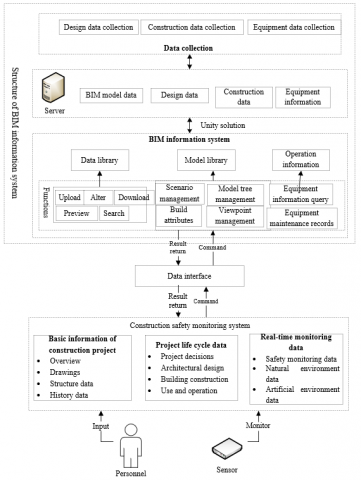Figure 3. Multi-sensor fusion data collection model based on the BIM information system

By generalizing construction safety issues, this paper built a construction safety monitoring data flow model, as shown in Figure 2. The input data of the monitoring data information layer includes basic information (such as sensor model, serial number, and parameters), real-time monitoring data information, and reference data values of monitoring positions.

In view of the complex building structure and natural and artificial environment, for a same safety issue, it is necessary to monitor it comprehensively using multiple indicators, at multiple positions and adopting sensors of multiple types. For construction safety monitoring, the commonly used monitoring sensors include load sensor, tilt angle sensor, displacement sensor, settlement sensor, crack sensor, temperature and humidity sensor, and sound and light alarms, etc.

However, when the real-time monitoring information of sensor network is used for the safety status evaluation of construction projects, misjudgments often occur, therefore, it requires detailed history data of each link in the construction cycle of the project to assist decision-making. Figure 3 shows the multi-sensor fusion data collection model based on the BIM information system.

3. The Spectrum Sensing Algorithm for Sensor Signals in Construction Area

Generally, the natural and artificial environment of construction projects is quite complex. In order to improve the performance of the construction safety monitoring system and its adaptability, lower the requirements for sensor hardware sensitivity, and realize the comprehensive monitoring of the same target by multiple types of sensors, it is necessary to explore the issues of spectrum sensing and classification detection of wireless sensor signals under different signal-to-noise ratios (SNRs). Figure 4 shows the multi-sensor deep fusion system built on physical and information systems.

## 4.pngFigure 4. Multi-sensor fusion system structure

Multiple types of sensors monitoring a same target can form a sensor cluster. In view of the spectrum sensing characteristics of sensors in the construction environment, in a sensor cluster within a region, assume there’re M core sensors and m assistant sensors, then for any sensor in the cluster, the regional WSN system model at sampling time t can be regarded as a binary model shown as Formula 1:

\left\{ \begin{align} & SM:o\left( t \right)=Noise\left( t \right) \\ & S{M}':o\left( t \right)=\sum\limits_{i=1}^{M}{{{s}_{i}}\left( t \right)+n\left( t \right)} \\ \end{align} \right.    (1)

where, SM is model without core sensor(s), and SM' is model with core sensor(s). si(t) is the cyclostationary signal of the sensor cluster in the zero-mean area without core sensor(s), Noise(t) is the zero-mean additive white Gaussian noise. Based on the model shown in Formula 1, the cyclic spectrum characteristics of the sensor signals in the case of SM and SM' were analyzed and the parameters were estimated. The corresponding eigenvectors can be expressed as F=(P, δs-max, δc-max)T and F'=(P', δ's-max, δ'c-max)T, respectively. P and P' respectively correspond to the average energy of the spectral function at the cycle frequency in the case of SM and SM'. δs-max and δ's-max are the spectral correlation coefficients in the case of SM and SM'. δc-max and δ'c-max correspond to the maximum value of the spectral function at c in the case of SM and SM'.

Assume: C is the cycle period and c=1/C is the cycle frequency. If the autocorrelation function A(t, λ) of the cyclic random signal o(t) of the sensor cluster in the zero-mean area shows periodic fluctuations, then it can be expressed by Formula 2:

${{A}^{c}}\left( \lambda \right)=\frac{1}{C}\int_{0}^{C}{A\left( t,\lambda \right)}{{e}^{-2\pi ctj}}dt$    (2)

The cyclic spectrum can be expressed as:

${{h}^{c}}\left( k \right)=\int_{-\infty }^{\infty }{{{A}^{c}}\left( \lambda \right)}{{e}^{-2\pi ctj}}dt$    (3)

In practical applications, the cyclic spectrum is often written in discrete form:

${{\hat{h}}^{c}}\left( k \right)=\frac{1}{LN}\sum\limits_{l=1}^{L}{O\left( {{t}_{l}},k+c/2 \right){{O}^{*}}}\left( {{t}_{l}},k-c/2 \right)$    (4)

The data signals collected by the sensors were divided into L segments according to the sampling time, and each segment had N sampling points. O(tl, k) is the discrete Fourier transform of o(t) of each time segment, and O*(tl, k) was the conjugate of O(tl, k). The average energy P of the eigenvector parameters can be calculated by Formula 5:

$P=\frac{1}{J}\sum\limits_{j=0}^{J-1}{{{\left| h\left( j \right) \right|}^{2}}}$    (5)

where, h(j) is the spectral function of ĥc(k) at c. The spectral correlation coefficients can be calculated by Formula 6:

$\delta _{o}^{c}\left( k \right)=\frac{{{h}^{c}}\left( k \right)}{\sqrt{h\left( k+c/2 \right)h\left( k-c/2 \right)}}$    (6)

The value range of the spectral correlation coefficients was [0,1], and the maximum value δs-max can be expressed by Formula 7:

${{\delta }_{s-\max }}=\max \left| \delta _{o}^{c}\left( k \right) \right|$    (7)

The maximum value δc-max of the spectral function at c can be expressed by Formula 8:

${{\delta }_{c-\max }}={{h}^{c}}\left( k \right)\left| _{c=1/C} \right.$    (8)

The eigenvector composed of the above parameters was taken as the training samples of the support vector machine (SVM) to perform the training, and the SVM that had completed the training can realize detection and classification of the samples, thereby achieving the spectrum sensing of the core sensor(s). Specific steps were as follows:

1) In case of core sensor(s), the X eigenvectors F'i=(P'i, δ'is-max, δ'ic-max)T were taken as the positive samples for SVM, wherein i=1, 2, …, X. In case of no core sensor, Y eigenvectors Fi=(Pi, δis-max, δic-max)T were taken as the negative samples for SVM.

2) Training set TS was constructed according to the positive and negative samples, and used to train the SVM.

3) Positive and negative samples were collected again to obtain the test sample set, and the trained SVM was used to perform classified test on the data signals collected by the core sensors.

Nc and Nf were assumed to be the numbers of samples that are classified correctly and incorrectly, the correct rate of detection of data collected by the core sensors can be expressed by Formula 9:

${{\phi }_{c}}=\frac{{{N}_{c}}}{{{N}_{c}}+{{N}_{f}}}$    (9)

4. Tempo-Spatial Correlation Analysis of Multi-sensor Monitoring Data

## 5.png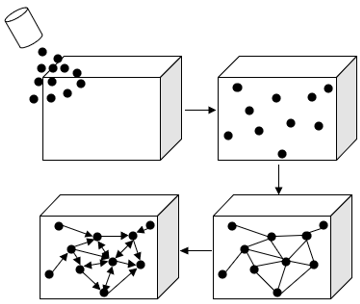Figure 5. Steps to generate wireless sensor construction safety monitoring network

Figure 5 gives the steps to generate the wireless sensor construction safety monitoring network. The sensors in the sensor cluster within the region have certain correlations. For a same sensor at a same monitoring point, the monitoring data obtained within a consecutive time period has certain temporal correlations. As for the multiple monitoring points in a region, the morning data obtained by sensors that are relatively close in the sensor cluster has certain spatial correlations.

In the safety monitoring process of construction projects, since the time interval of data sampling points was the same, the monitoring signals of each sensor in the unit sampling time period containing N sampling points can be regarded as a time series, which can be expressed as r(1), r(2),…, r(N). In the time series, if the covariance between any two adjacent monitoring data elements r(t) and r(t-n) is equal to zero, it indicates that the time series has no temporal correlation; if the said covariance is not equal to zero, the time series has temporal correlation. The n-order lag autocorrelation coefficient characterizing the degree of temporal correlation between r(t) and r(t-n) can be expressed by Formula 10:

${{R}_{n}}=\frac{\sum\limits_{t=n+1}^{N}{\left( {{r}_{t}}-\frac{1}{N}\sum\limits_{t=1}^{N}{{{r}_{i}}} \right)\left( {{r}_{t-n}}-\frac{1}{N}\sum\limits_{t=1}^{N}{{{r}_{i}}} \right)}}{\sum\limits_{t=1}^{N}{{{\left( {{r}_{t}}-\frac{1}{N}\sum\limits_{t=1}^{N}{{{r}_{i}}} \right)}^{2}}}}$    (10)

Since n is smaller than N, the value range of Rn was [0,1]. Under normal conditions, the basic characteristics of the sample data time series could be accurately obtained by calculating the autocorrelation coefficients of N/4 sampling points. If the autocorrelation coefficients of the entire time series are all approximately equal to 0, it can be considered that the sampling of the sensors within this time period is a random process, and the sample data sequence within this time period is a stationary time series. If Rn gradually decreases with the increase of n, and most of R1, R2,..., Rn are not approximately equal to 0, it can be considered that the sampling data of the sensors within this time period has obvious correlations, and the sample data sequence within this time period is a non-stationary time series.

For a given time series r(t-1), r(t-2),…, r(t-n-1), the degree of conditional correlation between r(t) and r(t-n) can be characterized by the partial correlation coefficient.

Assume Δn is the order of the lag of Rn, and total amount of sample data is N'. The LBQ statistics in the statistical tool Eviews can be expressed by Formula 11:

$LBQ={N}'\left( {N}'+2 \right)\sum\limits_{n=1}^{\Delta n}{\frac{R_{n}^{2}}{{N}'-n}}$   (11)

Taking monitoring points m1-m10 as examples, the temporal correlations of monitoring data at the same monitoring point in the construction project were analyzed. Table 1 shows the calculation of the time autocorrelation coefficients. According to the table, the 1-12 order autocorrelation coefficients of each monitoring point among m1-m10 all exceed 0 for a certain range, and it decreased gradually with the increase of n, which satisfied the condition of temporal correlations, and it can be proved that all 10 monitoring data time series had 12 order temporal correlations.

In terms of the spatial correlations of monitoring data, it is defined that as long as the sensors in the sensor cluster are close in space, then the monitoring data in a consecutive time period has spatial correlations, and it can be calculated by the Pearson formula shown as Formula 12:

$S=\frac{\sum\limits_{t=1}^{N}{{{m}_{i}}(t){{m}_{j}}(t)}-\frac{\sum\limits_{t=1}^{N}{{{m}_{i}}(t)\cdot \sum\limits_{t=1}^{N}{{{m}_{j}}(t)}}}{N}}{\sqrt{\left( \sum\limits_{t=1}^{N}{{{\left[ {{m}_{i}}(t) \right]}^{2}}}-\frac{{{\left[ \sum\limits_{t=1}^{N}{{{m}_{i}}(t)} \right]}^{2}}}{N} \right)\left( \sum\limits_{t=1}^{N}{{{\left[ {{m}_{j}}(t) \right]}^{2}}}-\frac{{{\left[ \sum\limits_{t=1}^{N}{{{m}_{j}}(t)} \right]}^{2}}}{N} \right)}}$    (12)

The value range of S is [-1,1]. The closer its value is to -1 or 1, the stronger the spatial correlations between the monitoring data of monitoring points mi and mj. When the value of S is closer to 0, the monitoring data of monitoring points mi and mj does not have spatial correlation. Taking monitoring points m1-m10 as examples, the spatial correlations of these monitoring points in the same area of a construction project were analyzed. Table 2 shows the calculation of the spatial autocorrelation coefficients. According to the table, the spatial correlation coefficient between each of the monitoring point m1-m10 and itself is 1, and the spatial correlation coefficients between each monitoring point with other monitoring points were about 0.9, which can prove that there are strong spatial correlations among the monitoring data of the 10 monitoring points.

Table 1. Temporal autocorrelation coefficients

 R1 R2 R3 R4 R5 R6 R7 R8 R9 R10 R11 R12 m1 0.921 0.882 0.795 0.621 0.676 0.587 0.577 0.476 0.384 0.321 0.253 0.196 m2 0.876 0.775 0.615 0.657 0.550 0.554 0.458 0.430 0.419 0.354 0.243 0.211 m3 0.911 0.890 0.765 0.666 0.680 0.511 0.594 0.521 0.432 0.435 0.361 0.210 m4 0.843 0.772 0.659 0.652 0.614 0.524 0.465 0.485 0.342 0.312 0.233 0.210 m5 0.878 0.798 0.698 0.598 0.576 0.584 0.498 0.435 0.324 0.310 0.300 0.278 m6 0.906 0.826 0.734 0.675 0.611 0.528 0.528 0.427 0.486 0.392 0.252 0.210 m7 0.842 0.719 0.677 0.523 0.487 0.498 0.346 0.387 0.375 0.254 0.222 0.201 m8 0.954 0.843 0.746 0.614 0.614 0.599 0.507 0.412 0.401 0.398 0.201 0.195 m9 0.897 0.775 0.645 0.523 0.476 0.423 0.332 0.350 0.329 0.289 0.268 0.241 m10 0.965 0.816 0.789 0.635 0.539 0.558 0.524 0.447 0.435 0.394 0.271 0.251

Table 2. Spatial autocorrelation coefficients

 m1 m2 m3 m4 m5 m6 m7 m8 m9 m10 m1 1.0000 0.9421 0.9579 0.9341 0.9261 0.9977 0.9425 0.9398 0.9574 0.9355 m2 0.9024 1.0000 0.9364 0.8954 0.8914 0.9216 0.9021 0.8936 0.8732 0.8321 m3 0.9498 0.9315 1.0000 0.9145 0.9874 0.9621 0.9456 0.9844 0.9574 0.9577 m4 0.9117 0.8975 0.9031 1.0000 0.8213 0.9458 0.8945 0.9271 0.9276 0.9227 m5 0.9217 0.8264 0.9147 0.8953 1.0000 0.9074 0.9402 0.9910 0.9225 0.9241 m6 0.9509 0.9401 0.9210 0.9459 0.9601 1.0000 0.9508 0.9677 0.9677 0.9907 m7 0.9147 0.8965 0.9245 0.9463 0.9745 0.9145 1.0000 0.9450 0.9211 0.9176 m8 0.9415 0.8456 0.9487 0.9951 0.9014 0.9166 0.9476 1.0000 0.9356 0.9546 m9 0.9750 0.8772 0.9493 0.9476 0.9257 0.9345 0.9210 0.8423 1.0000 0.9123 m10 0.9410 0.8560 0.9416 0.9744 0.9598 0.9750 0.9451 1.0000 0.8562 1.0000
5. MSMN-JS Model Construction and Missing Data Reconstruction

Due to the complexity of the natural and artificial environment of construction projects and the various ever-changing risk factors, some important monitoring points cannot install sensors, thus resulting in missing monitoring data. According to previous analysis, the monitoring signals within the construction area have strong tempo-spatial correlations, and they are sparsified under the discrete cosine basis. Therefore, based on this tempo-spatial correlation, it is possible to use distributed compressed sensing method to reconstruct the missing items of the monitoring data. Still, with monitoring points m1-m10 as examples, the sparse model was constructed; ξ represented the discrete cosine basis, then the monitoring data yi(g) of the i-th monitoring point at sampling time t can be expressed by Formula 13:

\left\{ \begin{align} & {{y}_{i}}=\xi \Lambda =\xi \left( {{\Lambda }_{p}}+{{\Lambda }_{i}} \right) \\ & {{\left\| {{\Lambda }_{p}} \right\|}_{0}}={{g}_{p}},{{\left\| {{\Lambda }_{i}} \right\|}_{0}}={{g}_{i}} \\ \end{align} \right.i\in \left\{ 1,2,\ldots ,\text{1}0 \right\}    (13)

In the formula, each yi containing two parts of valid monitoring data information ξΛp and invalid monitoring data information ξΛi could be expressed in sparse forms, gp and gi are the corresponding sparse coefficients. yi was sorted, since the valid monitoring data information ξΛp was public information, its sequence was the same with the original data sequence of yi, denoted as Γp. Then the missing data reconstruction problem can be equivalent to accurately recovering ξΛi according to existing Γp. Then equations were constructed for the complete monitoring data yi, as shown in Formula 14:

\left\{ \begin{align} & {{y}_{\text{1}}}=\xi \left( {{\Lambda }_{p}}+{{\Lambda }_{\text{1}}} \right) \\ & {{y}_{\text{2}}}=\xi \left( {{\Lambda }_{p}}+{{\Lambda }_{\text{2}}} \right) \\ & \vdots \\ & {{y}_{\text{10}}}=\xi \left( {{\Lambda }_{p}}+{{\Lambda }_{\text{10}}} \right) \\ \end{align} \right.    (14)

Its matrix form can be expressed by Formula 15:

$\left[\begin{array}{l}y_{1} \\ y_{2} \\ \vdots \\ y_{10}\end{array}\right]=\left[\begin{array}{ccccc}\xi & \xi & 0 & \cdots & 0 \\ \xi & 0 & \xi & \cdots & 0 \\ \vdots & \vdots & \vdots & \vdots & \vdots \\ \xi & 0 & 0 & \cdots & \xi\end{array}\right]\left[\begin{array}{l}\Lambda_{p} \\ \Lambda_{1} \\ \Lambda_{2} \\ \vdots \\ \Lambda_{10}\end{array}\right]$    (15)

The discrete cosine basis ξ was divided into two parts αi and βi respectively corresponding to the valid and invalid monitoring data information. The positions of elements of Γp in yi corresponded the positions of each row vector of αi in ξ one by one, then yi can be expressed as:

$\left\{\begin{array}{l}y_{i}=\left[\begin{array}{c}\Gamma_{p} \\ \Gamma_{i}\end{array}\right]=\left[\begin{array}{c}\alpha_{i} \\ \beta_{i}\end{array}\right]\left(\Lambda_{p}+\Lambda_{i}\right) \\ \left\|\Lambda_{p}\right\|_{0}=g_{p},\left\|\Lambda_{i}\right\|_{0}=g_{i}\end{array}\right.i \in\{1,2, \ldots, 10\}$    (16)

The valid monitoring data information Γp can be expressed by Formula 17:

\left\{ \begin{align} & {{\Gamma }_{p}}={{\alpha }_{i}}\left( {{\Lambda }_{p}}+{{\Lambda }_{i}} \right) \\ & {{\left\| {{\Lambda }_{p}} \right\|}_{0}}={{g}_{p}},{{\left\| {{\Lambda }_{i}} \right\|}_{0}}={{g}_{i}} \\ \end{align} \right.i\in \left\{ 1,2,\ldots ,10 \right\}    (17)

Simultaneous equations of Γp were constructed, as shown in Formula 18:

\left\{ \begin{align} & {{\Gamma }_{\text{1}}}={{\alpha }_{1}}\left( {{\Lambda }_{p}}+{{\Lambda }_{\text{1}}} \right) \\ & {{\Gamma }_{\text{2}}}={{\alpha }_{2}}\left( {{\Lambda }_{p}}+{{\Lambda }_{\text{2}}} \right) \\ & \vdots \text{ }\vdots \\ & {{\Gamma }_{\text{10}}}={{\alpha }_{10}}\left( {{\Lambda }_{p}}+{{\Lambda }_{\text{10}}} \right) \\ \end{align} \right.    (18)

Its matrix form can be expressed by Formula 19:

$\left[\begin{array}{l}\Gamma_{1} \\ \Gamma_{2} \\ \vdots \\ \Gamma_{10}\end{array}\right]=\left[\begin{array}{lllll}\alpha_{1} & \alpha_{1} & 0 & \cdots & 0 \\ \alpha_{2} & 0 & \alpha_{2} & \cdots & 0 \\ \vdots & \vdots & \vdots & \vdots & \vdots \\ \alpha_{10} & 0 & 0 & \cdots & \alpha_{10}\end{array}\right]\left[\begin{array}{l}\Lambda_{p} \\ \Lambda_{1} \\ \Lambda_{2} \\ \vdots \\ \Lambda_{10}\end{array}\right]$    (19)

$\Gamma=\left[\begin{array}{l}\Gamma_{1} \\ \Gamma_{2} \\ \vdots \\ \Gamma_{10}\end{array}\right], \alpha=\left[\begin{array}{lllll}\alpha_{1} & \alpha_{1} & 0 & \cdots & 0 \\ \alpha_{2} & 0 & \alpha_{2} & \cdots & 0 \\ \vdots & \vdots & \vdots & \vdots & \vdots \\ \alpha_{10} & 0 & 0 & \cdots & \alpha_{10}\end{array}\right], \Lambda=\left[\begin{array}{l}\Lambda_{p} \\ \Lambda_{1} \\ \Lambda_{2} \\ \vdots \\ \Lambda_{10}\end{array}\right]$

then, Formula 19 can be converted to:

\left\{ \begin{align} & \Gamma =\alpha \Lambda \\ & {{\left\| \Lambda \right\|}_{0}}=G \\ \end{align} \right.    (20)

The initial problem of missing data reconstruction can be transformed into:

$\min {{\left\| \vartheta \right\|}_{\text{0}}}s.t.y=A\vartheta$    (21)

The problem is a NP-hard problem that solves the number of non-zero elements in Λ, and using the L0 norm method to solve it will be too slow, therefore it was converted into the L1 norm convex programming problem shown in Formula 22:

$\min {{\left\| \vartheta \right\|}_{\text{1}}}s.t.y=A\vartheta$    (22)

The above L1 norm problem is usually transformed into:

$\underset{\vartheta }{\mathop{\min }}\,\left\| y-A\vartheta \right\|_{2}^{2}+\lambda {{\left\| \vartheta \right\|}_{1}}$    (23)

If the solution of Λ is obtained, then Λp and Λi can be obtained, and the complete monitoring data yi can be obtained by Formula 24:

${{y}_{i}}=\xi \left( {{\Lambda }_{p}}+{{\Lambda }_{i}} \right)$    (24)

6. Experimental Results and Analysis

In order to verify the correctness and reliability of the proposed algorithm for spectrum sensing of sensor signals in the complex natural and artificial environment of the construction area, this study designed simulation experiments for the two modulation signals: phase shift keying signals and frequency shift keying signals. In the simulation, the value range of the SNR was [-15, 5]. 1000 and 500 sets of data samples were randomly selected from the sensor monitoring database to construct the training set and test set, and the SVM and ANN models were trained and tested separately under 13 SNRs. Table 3 gives the correct rate of modulation signal spectrum sensing classification of the two algorithms and the proposed algorithm under two SNRs of -15dB and 5dB. According to the table, the correct rates of the three algorithms under 5dB SNR were all higher than those under -15dB SNR. Under the two SNRs, the correct rate of modulation signal spectrum sensing classification of the proposed algorithm was higher than those of the other two algorithms.

Table 3. Correct rate of regional sensor signal spectrum sensing of each algorithm

 Modulation signal type Correct rate (SNR = -5dB) Correct rate (SNR =5dB) ANN SVM The proposed algorithm ANN SVM The proposed algorithm Phase shift keying signal 57.61 72.45 86.47 81.40 89.40 90.31 Frequency shift keying signal 54.74 77.10 88.19 82.47 88.43 91.63

## 6.png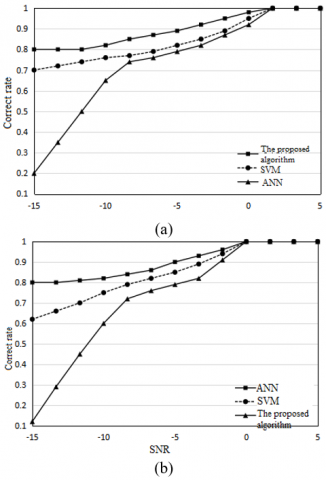Figure 6. Spectrum sensing effects of regional sensor signals of each algorithm under different modulation signals

## 7.png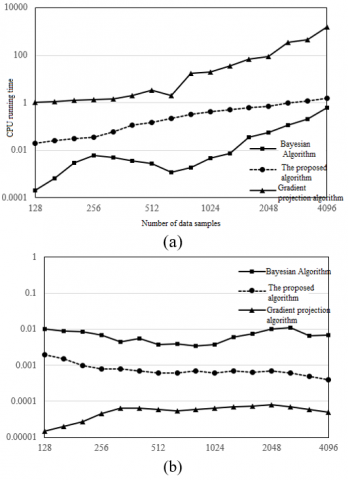Figure 7. CPU running time and NMSE of random signals

Figure 6 gives the regional sensor signal spectrum sensing effects of the three algorithms in the case of two modulation signals. Figure 6(a) corresponds to the phase shift keying signals, and Figure 6(b) corresponds to the frequency shift keying signals. It can be seen from the figures that, for the three algorithms, the correct rates were higher when SNR was greater than 0dB; when SNR was reduced, the correct rate of ANN greatly reduced; when the SNR was reduced to -15dB, the correct rate of ANN was only 21%, while for SVM and the proposed algorithm, this number was about 70% and 80.4%, respectively, the correct rate of the proposed algorithm was much higher than the other two algorithms.

## 8.png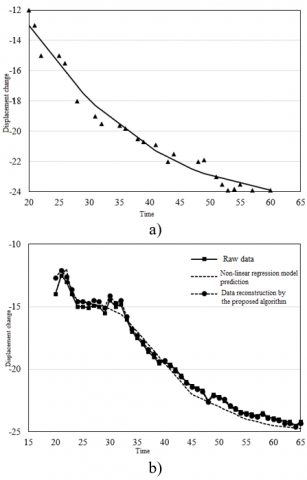Figure 8. Comparison of reconstruction effects of sensor signal missing data

In order to verify the reconstruction effects of the proposed algorithm on the missing data, this paper designed comparative experiments to compare the effects of the Bayesian algorithm, the gradient projection algorithm and the algorithm proposed in this paper. Figure 7 (a) and Figure 7 (b) respectively give the CPU running time spent by the three algorithms on random signal missing data reconstruction and their NMSE. According to the figures, the gradient projection algorithm had the fastest computation speed, but its error was larger, and the Bayesian algorithm was just the opposite. In comparison, the proposed algorithm showed the advantages of fast computation speed and small error. In view of the large data volume of the safety monitoring data of construction projects, and it requires the missing data reconstruction to achieve certain characterization, the proposed algorithm was an ideal choice for the problem of missing data reconstruction of safety monitoring of construction projects.

## 9a.png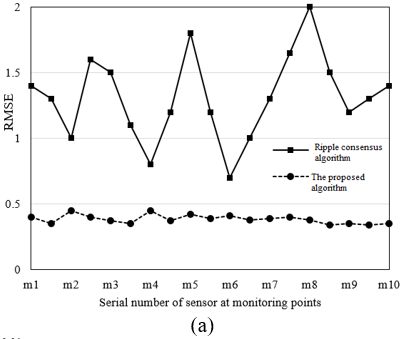## 9b.png## 9c.png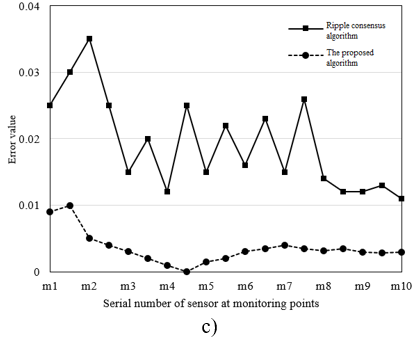Figure 9. Reconstruction effects of the proposed algorithm and Ripple consensus algorithm

In order to verify the advantages of the proposed algorithm in the reconstruction of missing monitoring data in actual construction projects, Figure 8 (a) gives the prediction results of the nonlinear regression model, and Figure 8 (b) gives the raw data, the prediction results of the nonlinear regression model, and the reconstruction results of the proposed algorithm. It can be seen from the figure, compared with the prediction curve of the nonlinear regression model, the reconstruction curve of the proposed algorithm was closer to the curve of raw data, and the degree of coincidence was higher.

The Ripple consensus algorithm is usually used to reconstruct low-rank matrices, it can effectively filter sparse noise of low-rank matrices containing missing data items. Since the signal data collected by the regional safety monitoring sensors of construction projects has large tempo-spatial correlations, therefore, the regional safety monitoring signals containing missing data items can be regarded as a damaged low-rank matrix, and this paper adopted the Ripple consensus algorithm to reconstruct it. Figure 9 shows the reconstruction effects of the proposed algorithm and the Ripple consensus algorithm.

According to the figure, the error value of the proposed algorithm in missing data reconstruction was much lower and that of the Ripple consensus algorithm, the fluctuation trend of the error curve of the proposed algorithm was more stable. This is because if the safety monitoring data of the construction projects contains a lot of Gaussian white noise, then it does not meet the requirements of the Ripple consensus algorithm for noise filtering conditions. Therefore, compared with the Ripple consensus algorithm, the proposed algorithm is more suitable for reconstructing the missing data of safety monitoring of construction projects. As for other noise types, Table 4 gives the calculation results of the errors of missing data reconstruction under the condition of sparse noise existing in the monitoring data, according to the table, when there’s large sparse noise in the monitoring data, the NMSEs of the proposed algorithm were all below 0.01, therefore, under the condition of different noise types, the reconstruction effect of the proposed algorithm was better. In conclusion, the reconstructed monitoring data can achieve effective evaluation of the safety status of construction projects.

Table 4. Missing data reconstruction errors of the proposed algorithm with sparse noise existing in the monitoring data

 m1 m2 m3 m4 m5 m6 m7 m8 m9 m10 RMSE 1.275 0.837 1.153 1.579 0.698 1.783 0.645 1.854 0.743 0.946 NMSE 0.0067 0.0038 0.0041 0.0027 0.0011 0.0079 0.0028 0.0054 0.0047 0.0069 Error value 0.061 0.027 0.019 0.045 0.072 0.079 0.029 0.018 0.049 0.056
7. Conclusion

This paper proposed a construction safety monitoring and evaluation model based on multi-sensor fusion. First, the system structure and data flow model of the construction safety monitoring system were constructed; then, combining with a multi-sensor deep fusion system which was built on physical and information systems, this paper designed a spectrum sensing algorithm for sensor signals in the construction area. By comparing the spectrum sensing effects of three algorithms on two modulation signals of phase shift keying signals and frequency shift keying signals under different SNRs, this paper proved that the proposed algorithm had higher correct rate in spectrum sensing classification. After that, based on the tempo-spatial correlation analysis of the multi-sensor monitoring data, this paper built a MSMN-JS model and realized the reconstruction of the missing monitoring data. At last, experimental results proved that the proposed algorithm had better reconstruction effects than other models under different noise conditions, and the research of this paper has certain application value for construction safety monitoring and evaluation.

Acknowledgment

This paper was supported by Natural Science Foundation of Shaanxi Province, China (Grant No.: 2019JQ-766), Natural Science Foundation of Shaanxi Educational Committee, China (Grant No.: 19JK0460), and Xi'an University of Architecture and Technology Research Grant, China (Grant No.: ZR19056).

References

 Martinez, J.G., Gheisari, M., Alarcón, L.F. (2020). UAV integration in current construction safety planning and monitoring processes: Case study of a high-rise building construction project in Chile. Journal of Management in Engineering, 36(3).

 Svintsov, A.P., Shchesnyak, E.L., Galishnikova, V.V., Fediuk, R.S. (2020). Monitoring of heating systems as a factor of energy safety of buildings. Journal of Building Engineering, 31: 101384. https://doi.org/10.1016/j.jobe.2020.101384

 Park, J., Cho, Y.K., Khodabandelu, A. (2018). Sensor-based safety performance evaluation of individual construction workers. Sensors (Switzerland), 18(11): 3897. https://doi.org/10.3390/s18113897

 Song, C., Wang, A., Lin, F., Xiao, J., Yao, X.W., Xu, W.Y. (2018). Selective CS: An energy-efficient sensing architecture for wireless implantable neural decoding. IEEE Journal on Emerging and Selected Topics in Circuits and Systems, 8(2): 201-210. https://doi.org/10.1109/JETCAS.2018.2809906

 Mangia, M., Bortolotti, D., Pareschi, F., Bartolini, A., Benini, L., Rovatti, R., Setti, G. (2017). Zeroing for HW-efficient compressed sensing architectures targeting data compression in wireless sensor networks. Microprocessors and Microsystems, 48: 69-79. https://doi.org/10.1016/j.micpro.2016.09.007

 Kim, Y., Park, J.S., Oh, B.K., Cho, T.J., Kim, J.M., Kim, S.H., Park, H.S. (2019). Practical wireless safety monitoring system of long-span girders subjected to construction loading a building under construction. Measurement: Journal of the International Measurement Confederation, 146: 524-536. https://doi.org/10.1016/j.measurement.2019.05.110

 Riaz, Z., Parn, E.A., Edwards, D.J., Arslan, M., Shen, C., Pena-Mora, F. (2017). BIM and sensor-based data management system for construction safety monitoring. Journal of Engineering, Design and Technology, 15(6): 738-753. https://doi.org/10.1108/JEDT-03-2017-0017

 Kelsey, C., Joseph, L. (2017). Leveraging telematics and real-time sensor data to increase safety of equipment-intensive construction operations. Proceedings, Annual Conference-Canadian Society for Civil Engineering, 2017: 328-336.

 Suguna, R., Rathinasabapathy, V. (2019). An SoC architecture for energy detection based spectrum sensing using Low Latency Column Bit Compressed (LLCBC) MAC in cognitive radio wireless sensor networks. Microprocessors and Microsystems, 69: 159-167. https://doi.org/10.1016/j.micpro.2019.06.005

 Lee, W.J., Cheon, M., Hyun, C.H., Park, M. (2013). Development of building fire safety system with automatic security firm monitoring capability. Fire Safety Journal, 58: 65-73. https://doi.org/10.1016/j.firesaf.2013.01.003

 Sita, I. (2012). Valentin.Building control, monitoring, safety and security using collaborative systems. Proceedings of the 2012 4th International Conference on Intelligent Networking and Collaborative Systems, INCoS 2012, pp. 662-667. https://doi.org/10.1109/iNCoS.2012.107

 Trutaev, S., Kuznetsov, K., Bykov, S., Juraido, B., Trutaeva, V. (2012). Development and implementation of Integrated Structural Health Monitoring Systems on Russia enterprises for ensuring safety operation of industrial equipment and buildings. 9th International Conference on Condition Monitoring and Machinery Failure Prevention Technologies, 2: 777-780.

 Teizer, J., Castro-Lacouture, D. (2007). Combined ultra-wideband positioning and range imaging sensing for productivity and safety monitoring in building construction. Congress on Computing in Civil Engineering, Proceedings, pp. 681-688.

 Sutton, F., Da Forno, R., Gschwend, D., Lim, R., Gsell, T., Beutel, J., Thiele, L. (2016). Poster abstract: A heterogeneous system architecture for event-triggered wireless sensing. 2016 15th ACM/IEEE International Conference on Information Processing in Sensor Networks, IPSN 2016-Proceedings. https://doi.org/10.1109/IPSN.2016.7460692

 Murty, M.S., Shrestha, R. (2016). VLSI architecture for cyclostationary feature detection based spectrum sensing for cognitive-radio wireless networks and its ASIC implementation. Proceedings of IEEE Computer Society Annual Symposium on VLSI, ISVLSI, 2016: 69-74. https://doi.org/10.1109/ISVLSI.2016.12

 Bellasi, D.E., Rovatti, R., Benini, L., Setti, G. (2015). A low-power architecture for punctured compressed sensing and estimation in wireless sensor-nodes. IEEE Transactions on Circuits and Systems I: Regular Papers, 62(5): 1296-1305. https://doi.org/10.1109/TCSI.2015.2418833

 Sukhanov, A.V., Prokof’ev, I.V., Ivanov, A.V. (2016). A universal digital platform for the construction of self-organizing wireless sensor networks for industrial safety and ecological monitoring systems. Russian Microelectronics, 45(2): 137-141. https://doi.org/10.1134/S1063739716020098

 Ryoo, B.Y., Chung, H.C. (2011). Wireless/mobile sensors for monitoring worker's health and safety in construction. Proceedings of the 28th International Symposium on Automation and Robotics in Construction, pp. 572-573.

 Joshi, N., Agarkhed, J. (2016). Path construction using cognitive radio sensing in wireless sensor network. Proceedings of IEEE International Conference on Circuit, Power and Computing Technologies. https://doi.org/10.1109/ICCPCT.2016.7530316

 Ozbey, B., Demir, H.V., Kurc, O., Erturk, V.B., Altintas, A. (2015). Wireless Sensing in Complex Electromagnetic Media: Construction Materials and Structural Monitoring. IEEE Sensors Journal, 15(10): 5545-5554.

 Taenaka, S., Watanabe, Y., Takayama, S. (2012). Construction of wireless sensing network system for landslide disaster monitoring. 20th IMEKO World Congress, 2: 955-960.

 Takai, H., Yasuda, G., Tachibana, K. (2002). Construction of infrared wireless inter-robot communication networks for distributed sensing and cooperation of multiple autonomous mobile robots. IFAC Proceedings Volumes (IFAC-PapersOnline), 15(1): 143-148. https://doi.org/10.3182/20020721-6-ES-1901.00930

 Schott, W., Gluhak, A., Presser, M., Hunkeler, U., Tafazolli, R. (2007). E-SENSE protocol stack architecture for wireless sensor networks. 2007 16th IST Mobile and Wireless Communications Summit, Budapest, Hungary, https://doi.org/10.1109/ISTMWC.2007.4299121